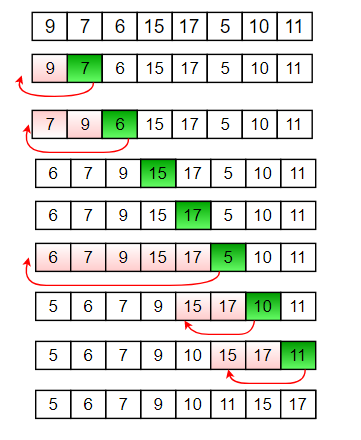# Recursive Insertion Sort

• Difficulty Level : Easy
• Last Updated : 28 Jul, 2022

Insertion sort is a simple sorting algorithm that works the way we sort playing cards in our hands.
Below is an iterative algorithm for insertion sort
Algorithm

```// Sort an arr[] of size n
insertionSort(arr, n)
Loop from i = 1 to n-1.
a) Pick element arr[i] and insert
it into sorted sequence arr[0..i-1] ```

Example:Refer Insertion Sort for more details.
How to implement it recursively?
Recursive Insertion Sort has no performance/implementation advantages, but can be a good question to check one’s understanding of Insertion Sort and recursion.
If we take a closer look at Insertion Sort algorithm, we keep processed elements sorted and insert new elements one by one in the sorted array.
Recursion Idea.

1. Base Case: If array size is 1 or smaller, return.
2. Recursively sort first n-1 elements.
3. Insert last element at its correct position in sorted array.

Below is implementation of above idea.

## C++

 `// Recursive C++ program for insertion sort``#include ``using` `namespace` `std;` `// Recursive function to sort an array using``// insertion sort``void` `insertionSortRecursive(``int` `arr[], ``int` `n)``{``    ``// Base case``    ``if` `(n <= 1)``        ``return``;` `    ``// Sort first n-1 elements``    ``insertionSortRecursive( arr, n-1 );` `    ``// Insert last element at its correct position``    ``// in sorted array.``    ``int` `last = arr[n-1];``    ``int` `j = n-2;` `    ``/* Move elements of arr[0..i-1], that are``    ``greater than key, to one position ahead``    ``of their current position */``    ``while` `(j >= 0 && arr[j] > last)``    ``{``        ``arr[j+1] = arr[j];``        ``j--;``    ``}``    ``arr[j+1] = last;``}` `// A utility function to print an array of size n``void` `printArray(``int` `arr[], ``int` `n)``{``    ``for` `(``int` `i=0; i < n; i++)``        ``cout << arr[i] <<``" "``;``}` `/* Driver program to test insertion sort */``int` `main()``{``    ``int` `arr[] = {12, 11, 13, 5, 6};``    ``int` `n = ``sizeof``(arr)/``sizeof``(arr);` `    ``insertionSortRecursive(arr, n);``    ``printArray(arr, n);` `    ``return` `0;``}`

## C

 `#include ` `// Recursive function to sort an array using``// insertion sort``void` `insertionSortRecursive(``int` `arr[], ``int` `n)``{``    ``// Base case``    ``if` `(n <= 1)``        ``return``;` `    ``// Sort first n-1 elements``    ``insertionSortRecursive(arr, n - 1);` `    ``// Insert last element at its correct position``    ``// in sorted array.``    ``int` `last = arr[n - 1];``    ``int` `j = n - 2;` `    ``/* Move elements of arr[0..i-1], that are``    ``greater than key, to one position ahead``    ``of their current position */``    ``while` `(j >= 0 && arr[j] > last) {``        ``arr[j + 1] = arr[j];``        ``j--;``    ``}``    ``arr[j + 1] = last;``}` `// A utility function to print an array of size n``void` `printArray(``int` `arr[], ``int` `size)``{``    ``int` `i;``    ``for` `(i = 0; i < size; i++)``        ``printf``(``"%d "``, arr[i]);``    ``printf``(``"\n"``);``}` `/* Driver program to test insertion sort */``int` `main()``{``    ``int` `arr[] = { 12, 11, 13, 5, 6 };``    ``int` `n = ``sizeof``(arr) / ``sizeof``(arr);` `    ``insertionSortRecursive(arr, n);``    ``printArray(arr, n);` `    ``return` `0;``}` `// This code is contributed by pariveshsrivastava1093`

## Java

 `// Recursive Java program for insertion sort` `import` `java.util.Arrays;` `public` `class` `GFG``{``    ``// Recursive function to sort an array using``    ``// insertion sort``    ``static` `void` `insertionSortRecursive(``int` `arr[], ``int` `n)``    ``{``        ``// Base case``        ``if` `(n <= ``1``)``            ``return``;``     ` `        ``// Sort first n-1 elements``        ``insertionSortRecursive( arr, n-``1` `);``     ` `        ``// Insert last element at its correct position``        ``// in sorted array.``        ``int` `last = arr[n-``1``];``        ``int` `j = n-``2``;``     ` `        ``/* Move elements of arr[0..i-1], that are``          ``greater than key, to one position ahead``          ``of their current position */``        ``while` `(j >= ``0` `&& arr[j] > last)``        ``{``            ``arr[j+``1``] = arr[j];``            ``j--;``        ``}``        ``arr[j+``1``] = last;``    ``}``    ` `    ``// Driver Method``    ``public` `static` `void` `main(String[] args)``    ``{``        ``int` `arr[] = {``12``, ``11``, ``13``, ``5``, ``6``};``     ` `        ``insertionSortRecursive(arr, arr.length);``        ` `        ``System.out.println(Arrays.toString(arr));``    ``}``}`

## Python3

 `# Recursive Python program for insertion sort``# Recursive function to sort an array using insertion sort` `def` `insertionSortRecursive(arr,n):``    ``# base case``    ``if` `n<``=``1``:``        ``return``    ` `    ``# Sort first n-1 elements``    ``insertionSortRecursive(arr,n``-``1``)``    ``'''Insert last element at its correct position``        ``in sorted array.'''``    ``last ``=` `arr[n``-``1``]``    ``j ``=` `n``-``2``    ` `      ``# Move elements of arr[0..i-1], that are``      ``# greater than key, to one position ahead``      ``# of their current position``    ``while` `(j>``=``0` `and` `arr[j]>last):``        ``arr[j``+``1``] ``=` `arr[j]``        ``j ``=` `j``-``1` `    ``arr[j``+``1``]``=``last``    ` `# A utility function to print an array of size n``def` `printArray(arr,n):``    ``for` `i ``in` `range``(n):``        ``print``(arr[i],end``=``" "``)` `# Driver program to test insertion sort``arr ``=` `[``12``,``11``,``13``,``5``,``6``]``n ``=` `len``(arr)``insertionSortRecursive(arr, n)``printArray(arr, n)` `# Contributed by Harsh Valecha`

## C#

 `// Recursive C# program``// for insertion sort``using` `System;` `class` `GFG``{` `    ``// Recursive function to sort``    ``// an array using insertion sort``    ``static` `void` `insertionSortRecursive(``int` `[]arr,``                                       ``int` `n)``    ``{``        ``// Base case``        ``if` `(n <= 1)``            ``return``;``    ` `        ``// Sort first n-1 elements``        ``insertionSortRecursive(arr, n - 1);``    ` `        ``// Insert last element at``        ``// its correct position``        ``// in sorted array.``        ``int` `last = arr[n - 1];``        ``int` `j = n - 2;``    ` `        ``/* Move elements of arr[0..i-1],``        ``that are greater than key, to``        ``one position ahead of their``        ``current position */``        ``while` `(j >= 0 && arr[j] > last)``        ``{``            ``arr[j + 1] = arr[j];``            ``j--;``        ``}``        ``arr[j + 1] = last;``    ``}``    ` `    ``//Driver Code``    ``static` `void` `Main()``    ``{``        ``int` `[]arr = {12, 11, 13, 5, 6};``    ` `        ``insertionSortRecursive(arr, arr.Length);``        ` `        ``for``(``int` `i = 0; i < arr.Length; i++)``        ``Console.Write(arr[i] + ``" "``);``    ``}``}` `// This code is contributed by Sam007`

## PHP

 `= 0 && ``\$arr``[``\$j``] > ``\$last``)``        ``{``            ``\$arr``[``\$j` `+ 1] = ``\$arr``[``\$j``];``            ``\$j``--;``        ``}``        ``\$arr``[``\$j` `+ 1] = ``\$last``;``    ``}``    ` `    ``// A utility function to``    ``// print an array of size n``    ``function` `printArray(&``\$arr``, ``\$n``)``    ``{``        ``for` `(``\$i` `= 0; ``\$i` `< ``\$n``; ``\$i``++)``            ``echo` `\$arr``[``\$i``].``" "``;``    ``}` `// Driver Code``\$arr` `= ``array``(12, 11, 13, 5, 6);``\$n` `= sizeof(``\$arr``);` `insertionSortRecursive(``\$arr``, ``\$n``);``printArray(``\$arr``, ``\$n``);` `// This code is contributed by ChitraNayal.``?>`

## Javascript

 ``

Output :

`5 6 11 12 13 `

Time Complexity: O(n2)
Auxiliary Space: O(n)